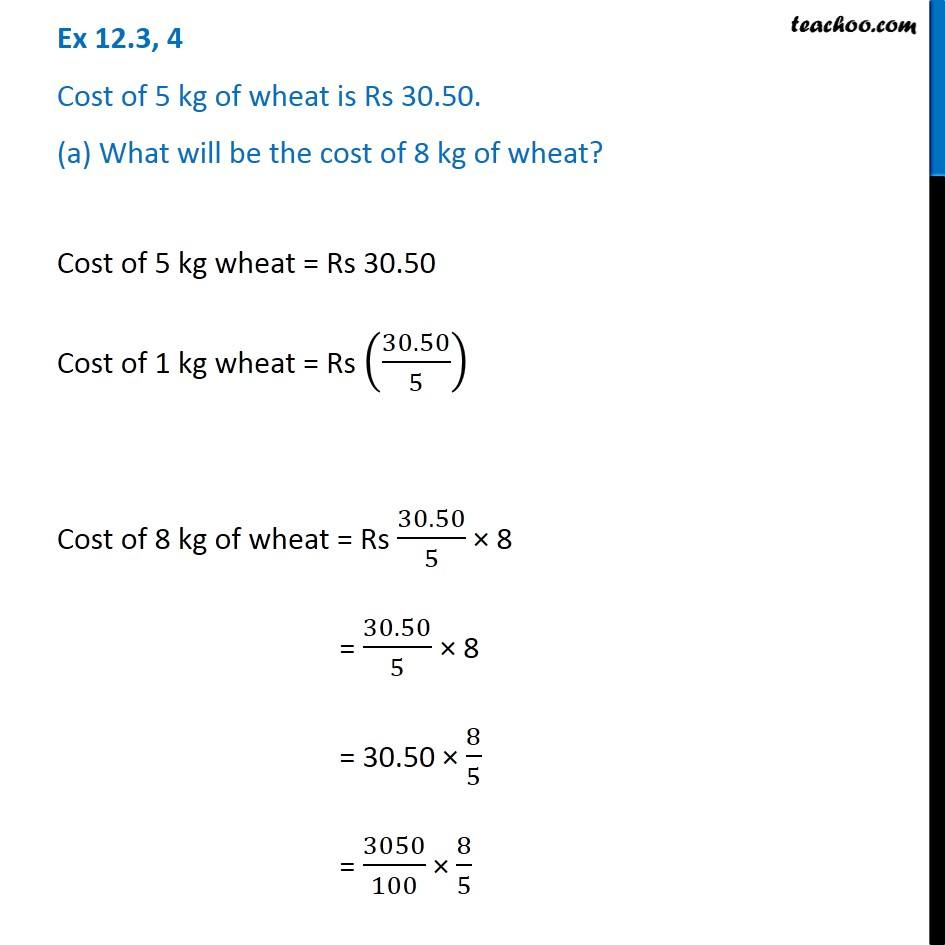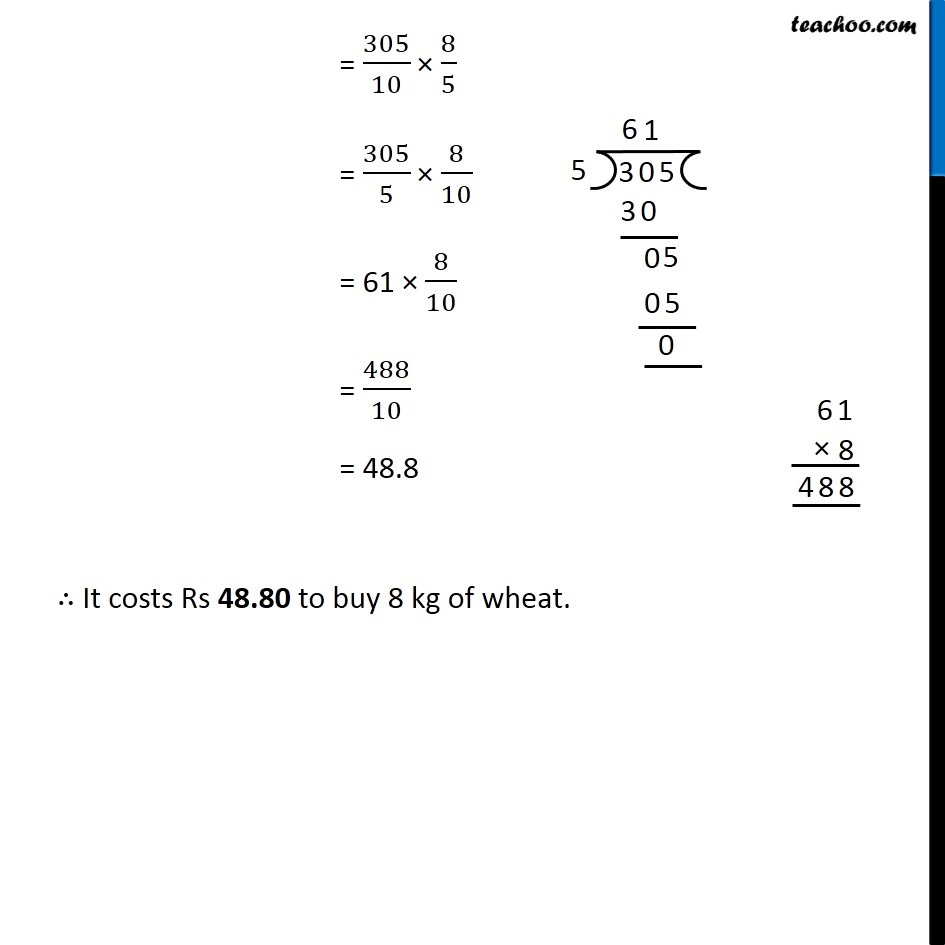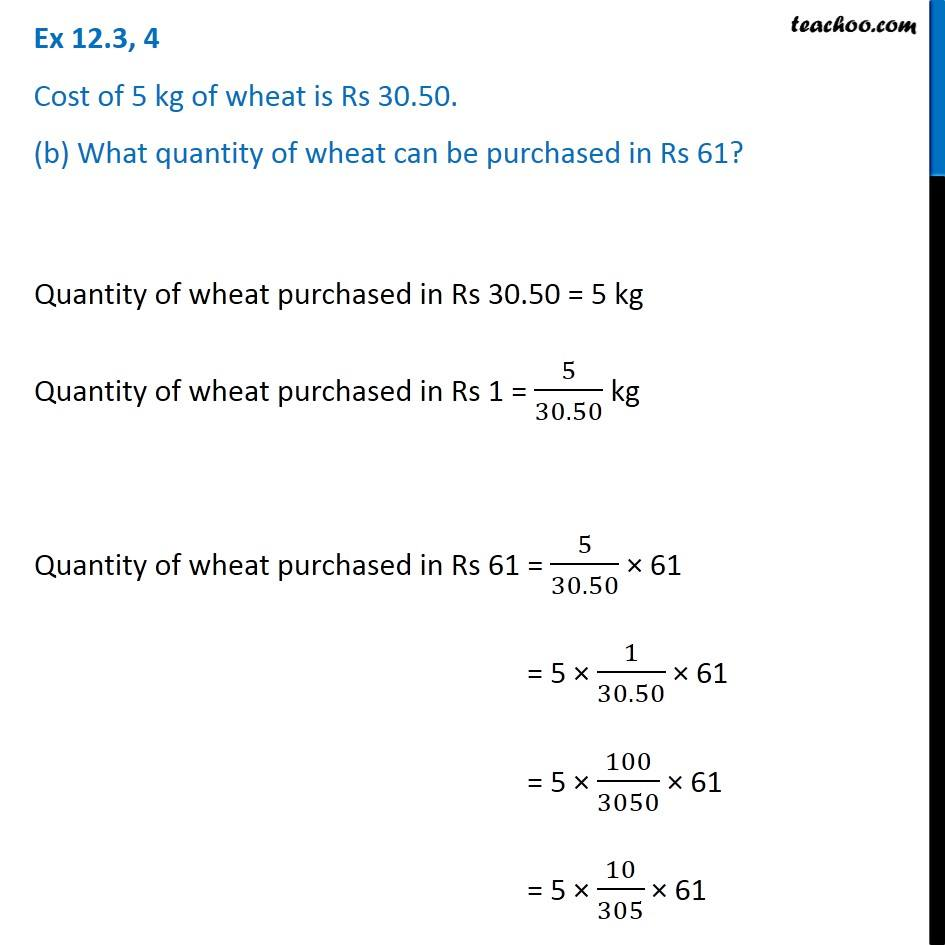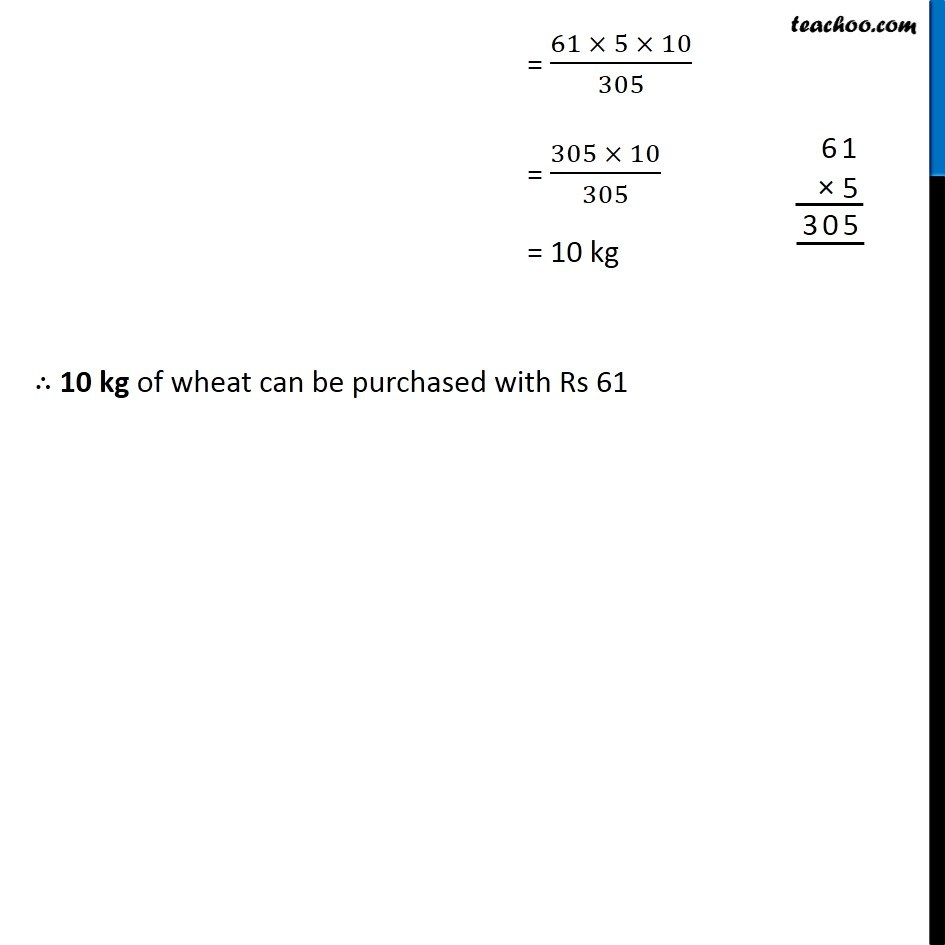Unitary Method

Chapter 12 Class 6 Ratio And Proportion
Concept wiseIntroducing your new favourite teacher - Teachoo Black, at only ₹83 per month

### Transcript

Ex 12.3, 4 Cost of 5 kg of wheat is Rs 30.50. (a) What will be the cost of 8 kg of wheat? Cost of 5 kg wheat = Rs 30.50 Cost of 1 kg wheat = Rs (30.50/5) Cost of 8 kg of wheat = Rs 30.50/5 × 8 = 30.50/5 × 8 = 30.50 × 8/5 = 3050/100 × 8/5 = 305/10 × 8/5 = 305/5 × 8/10 = 61 × 8/10 = 488/10 = 48.8 ∴ It costs Rs 48.80 to buy 8 kg of wheat. Ex 12.3, 4 Cost of 5 kg of wheat is Rs 30.50. (b) What quantity of wheat can be purchased in Rs 61?Quantity of wheat purchased in Rs 30.50 = 5 kg Quantity of wheat purchased in Rs 1 = 5/30.50 kg Quantity of wheat purchased in Rs 61 = 5/30.50 × 61 = 5 × 1/30.50 × 61 = 5 × 100/3050 × 61 = 5 × 10/305 × 61 = (61 × 5 × 10)/305 = (305 × 10)/305 = 10 kg ∴ 10 kg of wheat can be purchased with Rs 61